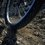# Brightness of light bulb

I was working through some questions on DC Circuits. I am confused as to on what factors does brightness of a light bulb depends.

For a bulb with given voltage and power, on what factors would its brightness depend? Suppose we increase the voltage more than labeled on it; would the brightness decrease, increase or remain the same? Likewise, what would happen if we decrease the voltage?

I am totally confused regarding the factors on which brightness of bulb depends. Further why do we use different bulbs if brighness of a bulb can be controlled. I would be grateful if someone could explain this or provide an online source relevant to the understanding of this topic.

Bulb 1 is labelled as 60W, 120V and bulb 2 is labelled as 120W, 120V. Which one would be more bright when connected in series and parallel?

This is what I think about the problem:

The resistance of both bulbs are respectively, 240 ohm and 120 ohm. Since the current is same in series. The power dissipated would be more in bulb 1. Bulb 1 would be more bright in this case. When the bulbs are connected in parallel, the power dissipated would be more in bulb 2. Bulb 2 will more bright in this case.Note by Lokesh Sharma
7 years, 8 months ago

This discussion board is a place to discuss our Daily Challenges and the math and science related to those challenges. Explanations are more than just a solution — they should explain the steps and thinking strategies that you used to obtain the solution. Comments should further the discussion of math and science.

When posting on Brilliant:

• Use the emojis to react to an explanation, whether you're congratulating a job well done , or just really confused .
• Ask specific questions about the challenge or the steps in somebody's explanation. Well-posed questions can add a lot to the discussion, but posting "I don't understand!" doesn't help anyone.
• Try to contribute something new to the discussion, whether it is an extension, generalization or other idea related to the challenge.

MarkdownAppears as
*italics* or _italics_ italics
**bold** or __bold__ bold
- bulleted- list
• bulleted
• list
1. numbered2. list
1. numbered
2. list
Note: you must add a full line of space before and after lists for them to show up correctly
paragraph 1paragraph 2

paragraph 1

paragraph 2

[example link](https://brilliant.org)example link
> This is a quote
This is a quote
    # I indented these lines
# 4 spaces, and now they show
# up as a code block.

print "hello world"
# I indented these lines
# 4 spaces, and now they show
# up as a code block.

print "hello world"
MathAppears as
Remember to wrap math in $$ ... $$ or $ ... $ to ensure proper formatting.
2 \times 3 $2 \times 3$
2^{34} $2^{34}$
a_{i-1} $a_{i-1}$
\frac{2}{3} $\frac{2}{3}$
\sqrt{2} $\sqrt{2}$
\sum_{i=1}^3 $\sum_{i=1}^3$
\sin \theta $\sin \theta$
\boxed{123} $\boxed{123}$

Sort by:

Its correct

- 7 years, 3 months ago

I think voltage doesn't necessarily correlate with the brightness of a bulb. This can be proved as follows: We get some bulbs of different ratings such that their resistances are different.On connecting them parallel to a battery,they take up the same voltage but their brightness differs.

- 7 years, 8 months ago

Okay... Thanks

- 7 years, 8 months ago

- 2 years, 12 months ago

if you increase the voltage over the "recommended" it will blow the filament P=VI so if you increase the voltage you increase the voltage or current or decrease the resistance the power will increase therefor the light will be brighter. for the example when it saws 120v, 120w its saying when there is 120 volts running through the light the power out put is 120w(it does not mean it is running at that amount it could be less). say you have a power supply that has a voltage of 120v when in series the voltage is divided(unevenly) the one with the most most v would be the brightest. in parallel its a bit easier the 120W bulb would be brighter since '120V" is running through each branch.

- 7 years, 8 months ago